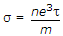# Materials and Components – Section 5

21.  The dipole moment per unit volume P as a function of E for an insulator is
 A. P = ∈0 E(∈r) B. P = ∈0 E C. P = ∈0(∈r – 1) E D. P =22.  A good dielectric should have
 A. low losses B. good heat conductivity C. high intrinsic strength D. all of the above

23.  Magnetic hysteresis phenomenon is explained by
 A. motion of domain walls B. motion of domain walls and domain rotation C. domain rotation D. none of the above

24.  If a metal specimen has n valence electrons per m3 each having charge e and mass m and t is the relaxations time, the conductivity is
 A.B.C.D.25.  The relation between thermal conductivity K (W/m/°C), heat flow density Q (watt/m2) and dT/dx (°C per metre) is
 A. Q = K(dT/dx) B. Q = – K(dT/dx) C. K = Q(dT/dx) D. dT/dx = – QK

26.  As per Matthiessen’s rule, the total resistivity r of a conductor can be written as r = ri + aT, where ri and a are constants and T is absolute temperature. At very low temperatures
 A. aT = 0 B. aT > ri C. aT ∼ ri D. aT < ri

Explanation:

At very low temperatures a T → 0. Since resistivity has a finite value even at low temperature, a T < ri.

27.  Consider the following statement: In case of a superconductor

1. B = 0
2. μr is high
3. diamagnetism is high
4. transition temperature varies with isotopic mass.

Of the above statements

 A. 1 and 2 are correct B. 2, 3, 4 are correct C. 1, 3, 4 are correct D. 2, 4 are correct

28. Assertion (A): In liquids and glassy substances, dielectric losses occur.

Reason (R): The orientation polarization in many substances is frequency dependent.

 A. Both A and R are true and R is correct explanation of A B. Both A and R are true but R is not correct explanation of A C. A is true but R is false D. A is false but R is true

29.  A single layer air cored coil has n turns and inductance L. If a new coil is formed by having 2n turns while keeping the coil length and diameter the same, the new inductance is
 A. 0.5 L B. L C. 2 L D. 4 L

Explanation:

Inductance is proportional to square of number of turns.

30.  The colour code on a carbon resistor is yellow-violet-orange. The value of resistance is
 A. 17000 Ω B. 27000 Ω C. 37000 Ω D. 47000 Ω